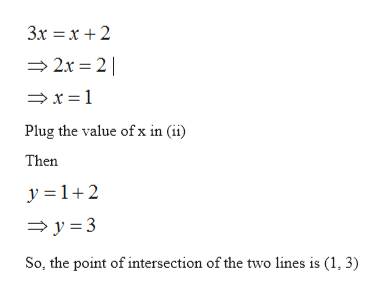Question
7 views
check_circle

Step 1

given equations of lines are

Step 2

By arranging the terms of equation (i)

Step 3

Graphical solution of the lines is the point of intersection of those lines

So, plot the lines (iii) and (ii) on the graph ...help_outlineImage Transcriptionclose3x x2 2x 2 x=1 Plug the value of x in (ii) Then y 1+2 y 3 So, the point of intersection of the two lines is (1, 3) fullscreen

### Want to see the full answer?

See Solution

#### Want to see this answer and more?

Solutions are written by subject experts who are available 24/7. Questions are typically answered within 1 hour.*

See Solution
*Response times may vary by subject and question.
Tagged in

### Linear Programming